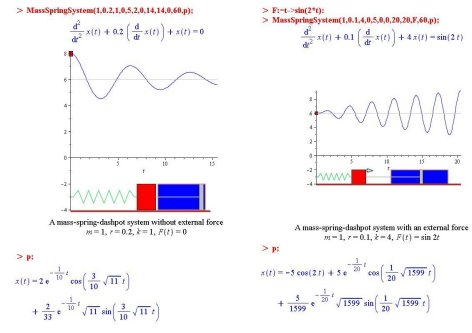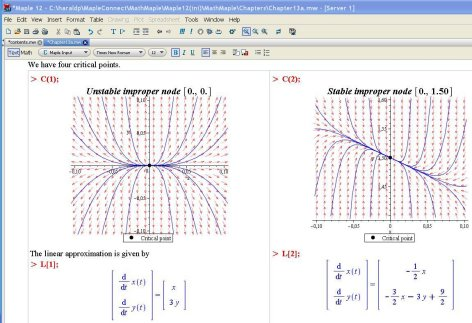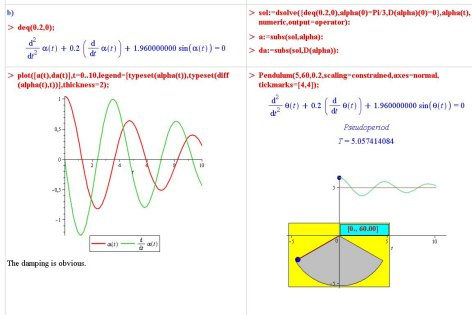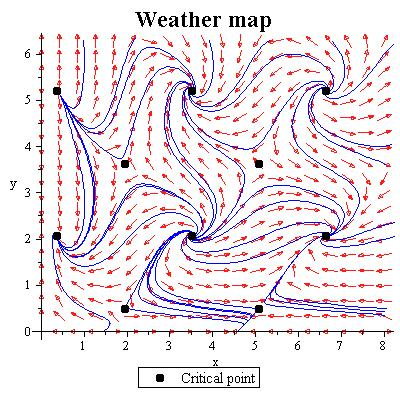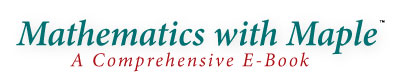Product Description

Mathematics with Maple is a comprehensive, interactive and highly visual electronic textbook written in Maple. This e-book consists of 22 chapters that cover more than 100 topics from mathematics, applied mathematics, and statistics at the university level. It includes more than 600 worked examples, over 140 new commands for learning and visualizing concepts from calculus, and summaries of the mathematical concepts and principles associated with each topic. This unique material can be used as a supplement to a standard textbook or on its own.

The main objective of this e-book is to show how Maple can be integrated as a valuable and powerful tool for students and educators. The material allow users to focus on concepts and examples to get a better understanding of the mathematics rather than spending time on the details of the calculations.

This e-book gives teachers a useful resource as a computational supplement for courses in mathematics, applied mathematics, science, engineering, and statistics, and allows for easy, highly visual classroom demonstrations. For students, this e-book provides help, motivation, and insight into theory and applications. The computations and computational experience gained from this book can be used in classes, labs, homework, projects, and also in engineering and other professions.

 Intended Audience
 Mathematics with Maple will be of interest to Students who are studying mathematics, science, or engineering at the university level, especially those who would like to learn how to make effective use of Maple in classes, labs, homework, projects, and future employment Educators teaching mathematics, science, or engineering at the university level who are interested in using Maple as a visualization and computational tool in the classroom
 Key Features
• This e-book is a collection of Maple worksheets covering more than 100 topics from mathematics, applied mathematics, and statistics at the university level.
• The over 600 varied and realistic examples can be modified and reused.
• Organized into 22 chapters, this e-book is the equivalent of approximately 2500 printed pages of content.
• Each topic includes a summary of the mathematical concepts and principles, allowing this book to be used as a textbook on its own, as well as a supplementary text.
• This product includes two new Maple library packages for understanding and visualizing concepts from introductory and advanced calculus.
• 180 commands allow you to perform complex operations in Maple without the need to understand the subtleties of Maple's plotting and other base commands.
• Includes a very wide variety of commands, covering such diverse topics as heat equations, Bezier curves, line integrals, truth tables, Pascal's triangle, and statistical procedures which can replace many of the statistical tables found in standard textbooks.
• Commands can be used with the e-book content and in your own worksheets.
• Each command is fully documented using standard Maple help pages.
• Multiple approaches are used. This book shows how to use Maple as a computational tool to obtain immediate solutions and it uses Maple to solve problems step-by-step the way a traditional textbook would.
• All Maple commands used in the worksheets are hyperlinked to the corresponding help pages to provide easy access to on-line help at any time during a session.
 The content includes: Functions and graphs Limits, derivatives, integration and multiple integration Complex numbers, linear algebra Ordinary and partial differential equations Conics, polar coordinates, parametric curves Curves and surfaces in space, multivariable functions Vectors and vector analysis Integral transforms, numerical methods, linear optimization Logic, combinatoric and statistics Conformal mappings, graphs and trees View the full Table of Contents